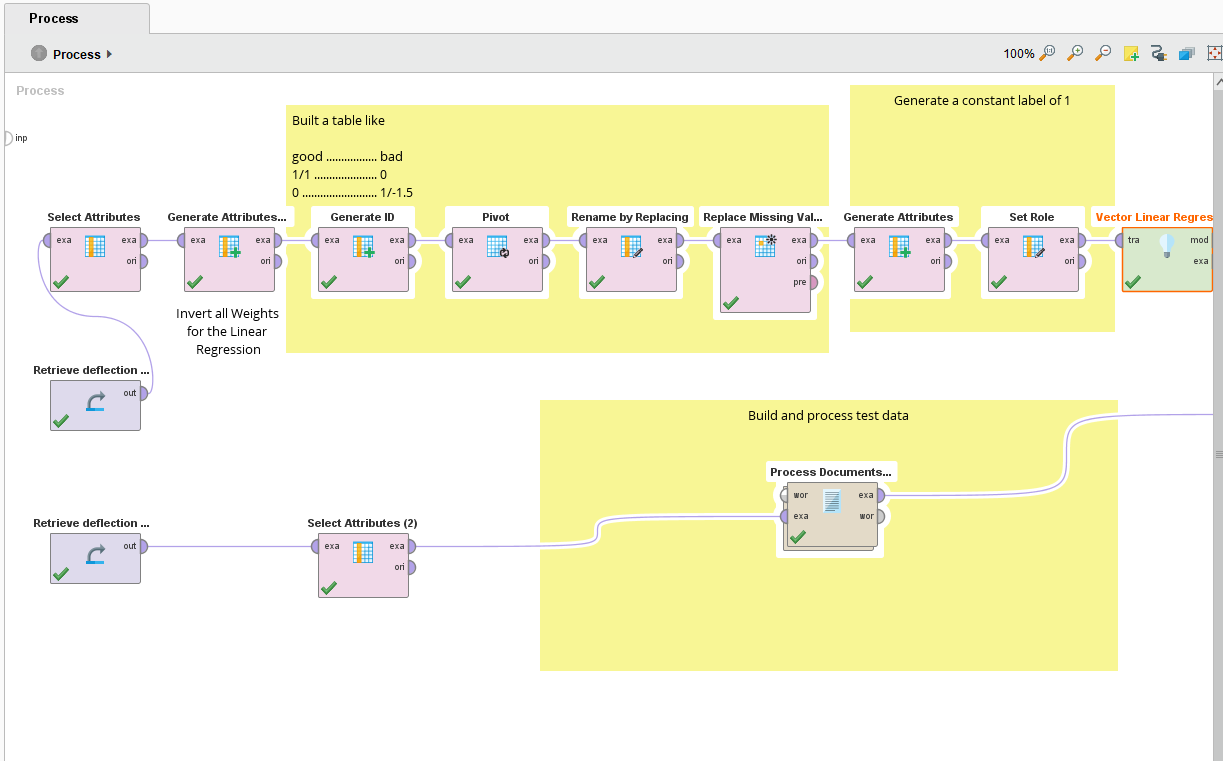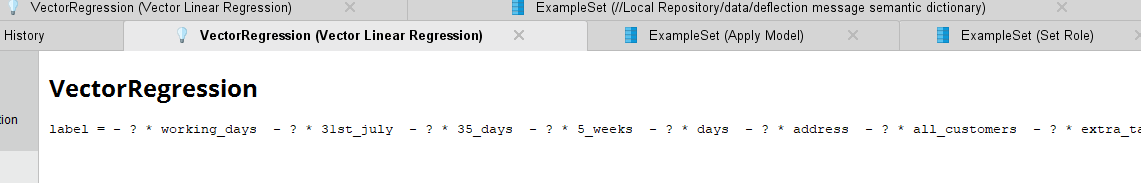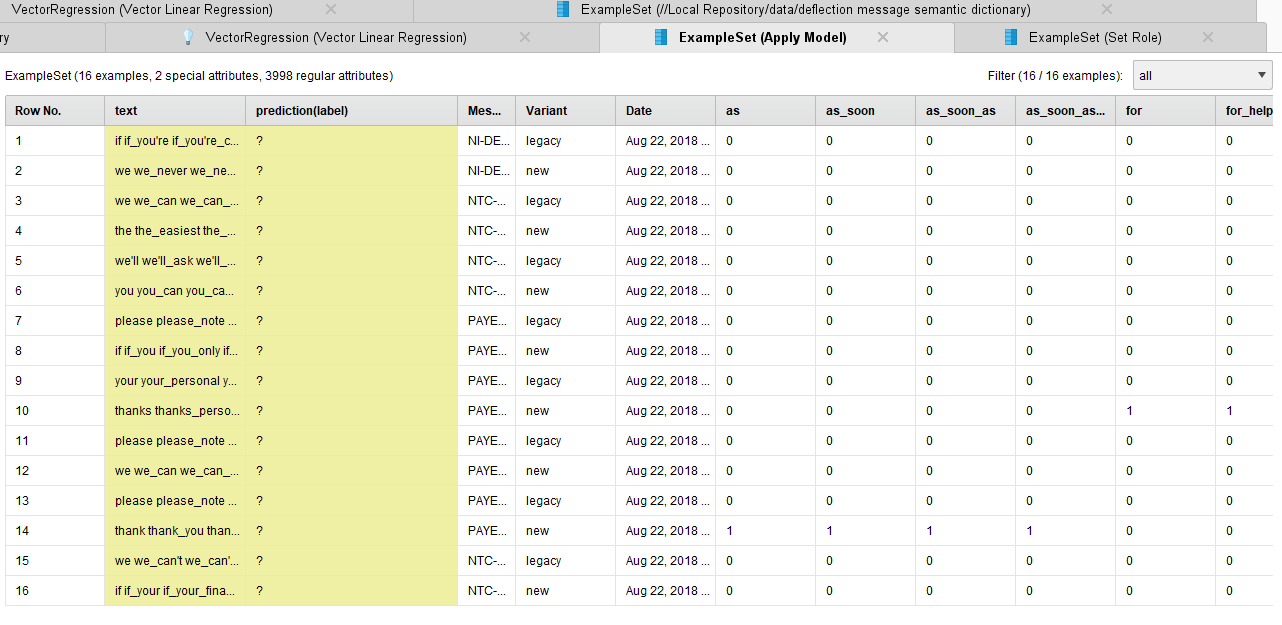# Defeated by a simple text analysis problem - user defined sentiment dictionary

Member Posts: 5Contributor I
edited December 2018 in Help

Hello all, I'm new here and to rapidminer so please be gentle.

I have been defeated by what seems like a simple text analysis problem - pretty much a word counting task - and spent a whole day on it. I have googled and looked through the forums to find an answer, but am still stuck.   I figured I could do this in PERL, but it would be quicker in RapidMiner - but I guess I have been caught by the learning curve.

The task seems simple to me. I wish to load some text (for now one "document" will do, although I actually have about 10, each comprising around a hundred words)..    Within that text I wish to identify some words/phrases as a "+1" and some others as "-1" score. I want to count the occurence of these words in the document and generate two numbers: A: the number of unique occurences and B: the sum of their scores.  (What I ultimately want to calculate is a clarity score for the text, so these dictionary scores indicate whether a word aids or hinders clarity)

This seemed ever so easy and I turned to initally to the Dictionary Based Sentiment operator. Built my process, but there is a problem using the "Apply" operator for DBS - namely it is "whited out" and says "deprecated" in the notes. It will not run. Seems like this would have been the perfect solution, but I can't use it.

So, I turned to the next solution as listed in the forum here: RapidMiner-Studio-Knowledge-Base/How-to-Build-a-Dictionary-Based-Sentiment-Model-in-RapidMiner/

I got the example working, so started to modify the inputs to use my source data and dictionary:The first problem I hit was the same as the user at the bottom of the above page: namely that if you add to the dictionary and your dictionary term is not actually in the source text, the Apply Model operator will not run, as it expects the dictionary to be a subset. (Which seems odd, since in the general sense a dictionary is a superset of all possible definitions).  Anyway, I fixed that by laboriously editing down my dictionary against each source document.. (So already negated the purpose of trying to automate this!)

The process now runs, but the next problem is, theVector Linear Regression model does not calculate any values!  In fact I just see this in its output (a bunch of ? instead of the scores from the dictionary):and, of course, in the results the "prediction" (which all I want to be the sum of the "sentiment" values) is a bunch of "?'s"I've tried for hours to figure out what is going wrong and why this won't work...  is there a way to get this to work, or a simpler method, that would be as simple as using the "Dictionary Based Sentiment" operator?

Many thanks

nik

`<?xml version="1.0" encoding="UTF-8"?><process version="9.0.001">  <context>    <input/>    <output/>    <macros/>  </context>  <operator activated="true" class="process" compatibility="9.0.001" expanded="true" name="Process">    <process expanded="true">      <operator activated="true" class="retrieve" compatibility="9.0.001" expanded="true" height="68" name="Retrieve deflection message semantic dictionary" width="90" x="45" y="289">        <parameter key="repository_entry" value="../data/deflection message semantic dictionary"/>      </operator>      <operator activated="true" class="select_attributes" compatibility="9.0.001" expanded="true" height="82" name="Select Attributes" width="90" x="45" y="136"/>      <operator activated="true" class="generate_attributes" compatibility="9.0.001" expanded="true" height="82" name="Generate Attributes (2)" width="90" x="179" y="136">        <list key="function_descriptions">          <parameter key="Weight" value="1/Weight"/>        </list>        <description align="center" color="transparent" colored="false" width="126">Invert all Weights for the Linear Regression</description>      </operator>      <operator activated="true" class="generate_id" compatibility="9.0.001" expanded="true" height="82" name="Generate ID" width="90" x="313" y="136"/>      <operator activated="true" class="pivot" compatibility="9.0.001" expanded="true" height="82" name="Pivot" width="90" x="447" y="136">        <parameter key="group_attribute" value="id"/>        <parameter key="index_attribute" value="phrase_ngram"/>        <parameter key="skip_constant_attributes" value="false"/>      </operator>      <operator activated="true" class="rename_by_replacing" compatibility="9.0.001" expanded="true" height="82" name="Rename by Replacing" width="90" x="581" y="136">        <parameter key="replace_what" value="Weight_(.+)"/>        <parameter key="replace_by" value="\$1"/>      </operator>      <operator activated="true" class="replace_missing_values" compatibility="9.0.001" expanded="true" height="103" name="Replace Missing Values" width="90" x="715" y="136">        <parameter key="default" value="zero"/>        <list key="columns"/>      </operator>      <operator activated="true" class="generate_attributes" compatibility="9.0.001" expanded="true" height="82" name="Generate Attributes" width="90" x="849" y="136">        <list key="function_descriptions">          <parameter key="label" value="1"/>        </list>      </operator>      <operator activated="true" class="set_role" compatibility="9.0.001" expanded="true" height="82" name="Set Role" width="90" x="983" y="136">        <parameter key="attribute_name" value="label"/>        <parameter key="target_role" value="label"/>        <list key="set_additional_roles"/>      </operator>      <operator activated="true" class="vector_linear_regression" compatibility="9.0.001" expanded="true" height="82" name="Vector Linear Regression" width="90" x="1117" y="136">        <parameter key="use_bias" value="false"/>      </operator>      <operator activated="true" class="retrieve" compatibility="9.0.001" expanded="true" height="68" name="Retrieve deflection message content OR10 ABCD" width="90" x="45" y="442">        <parameter key="repository_entry" value="../data/deflection message content OR10 ABCD"/>      </operator>      <operator activated="true" class="select_attributes" compatibility="9.0.001" expanded="true" height="82" name="Select Attributes (2)" width="90" x="313" y="442"/>      <operator activated="true" class="text:process_document_from_data" compatibility="8.1.000" expanded="true" height="82" name="Process Documents from Data" width="90" x="782" y="391">        <parameter key="vector_creation" value="Term Occurrences"/>        <parameter key="keep_text" value="true"/>        <list key="specify_weights"/>        <process expanded="true">          <operator activated="true" class="text:tokenize" compatibility="8.1.000" expanded="true" height="68" name="Tokenize" width="90" x="179" y="34">            <parameter key="mode" value="specify characters"/>            <parameter key="characters" value=".: ,-"/>          </operator>          <operator activated="true" class="text:transform_cases" compatibility="8.1.000" expanded="true" height="68" name="Transform Cases" width="90" x="380" y="34"/>          <operator activated="true" class="text:generate_n_grams_terms" compatibility="8.1.000" expanded="true" height="68" name="Generate n-Grams (Terms)" width="90" x="581" y="34">            <parameter key="max_length" value="4"/>          </operator>          <connect from_port="document" to_op="Tokenize" to_port="document"/>          <connect from_op="Tokenize" from_port="document" to_op="Transform Cases" to_port="document"/>          <connect from_op="Transform Cases" from_port="document" to_op="Generate n-Grams (Terms)" to_port="document"/>          <connect from_op="Generate n-Grams (Terms)" from_port="document" to_port="document 1"/>          <portSpacing port="source_document" spacing="0"/>          <portSpacing port="sink_document 1" spacing="0"/>          <portSpacing port="sink_document 2" spacing="0"/>        </process>      </operator>      <operator activated="true" class="apply_model" compatibility="9.0.001" expanded="true" height="82" name="Apply Model" width="90" x="1251" y="289">        <list key="application_parameters"/>      </operator>      <connect from_op="Retrieve deflection message semantic dictionary" from_port="output" to_op="Select Attributes" to_port="example set input"/>      <connect from_op="Select Attributes" from_port="example set output" to_op="Generate Attributes (2)" to_port="example set input"/>      <connect from_op="Generate Attributes (2)" from_port="example set output" to_op="Generate ID" to_port="example set input"/>      <connect from_op="Generate ID" from_port="example set output" to_op="Pivot" to_port="example set input"/>      <connect from_op="Pivot" from_port="example set output" to_op="Rename by Replacing" to_port="example set input"/>      <connect from_op="Rename by Replacing" from_port="example set output" to_op="Replace Missing Values" to_port="example set input"/>      <connect from_op="Replace Missing Values" from_port="example set output" to_op="Generate Attributes" to_port="example set input"/>      <connect from_op="Generate Attributes" from_port="example set output" to_op="Set Role" to_port="example set input"/>      <connect from_op="Set Role" from_port="example set output" to_op="Vector Linear Regression" to_port="training set"/>      <connect from_op="Vector Linear Regression" from_port="model" to_op="Apply Model" to_port="model"/>      <connect from_op="Vector Linear Regression" from_port="exampleSet" to_port="result 1"/>      <connect from_op="Retrieve deflection message content OR10 ABCD" from_port="output" to_op="Select Attributes (2)" to_port="example set input"/>      <connect from_op="Select Attributes (2)" from_port="example set output" to_op="Process Documents from Data" to_port="example set"/>      <connect from_op="Process Documents from Data" from_port="example set" to_op="Apply Model" to_port="unlabelled data"/>      <connect from_op="Apply Model" from_port="labelled data" to_port="result 2"/>      <connect from_op="Apply Model" from_port="model" to_port="result 3"/>      <portSpacing port="source_input 1" spacing="0"/>      <portSpacing port="sink_result 1" spacing="0"/>      <portSpacing port="sink_result 2" spacing="0"/>      <portSpacing port="sink_result 3" spacing="0"/>      <portSpacing port="sink_result 4" spacing="0"/>      <description align="left" color="yellow" colored="false" height="248" resized="true" width="543" x="281" y="32">Built a table like&lt;br&gt;&lt;br&gt;good ................. bad&lt;br&gt;1/1 ..................... 0&lt;br&gt;0 ......................... 1/-1.5</description>      <description align="center" color="yellow" colored="false" height="247" resized="true" width="265" x="845" y="12">Generate a constant label of 1</description>      <description align="center" color="yellow" colored="false" height="271" resized="true" width="578" x="535" y="327">Build and process test data</description>    </process>  </operator></process>`

• Moderator, RapidMiner Certified Analyst, RapidMiner Certified Expert, Member Posts: 1,635Unicorn

This definitely looks like one for @mschmitz since he is the creator of the latest DBSM.

But possibly your first problem (words present in the document but not in the dictionary) could be addressed via automation by first processing all your documents into a single corpus and then creating a unified wordlist from that.  You can then use that wordlist to create your dictionary (and just leave the score of zero for words that you don't care about).  You should then be able to use that same wordlist when processing all future texts (there is a wordlist import on the Process Documents operator specifically for this purpose), so you would never end up with a mismatch between the wordlist for the documents you want to score vs what was in your dictionary (until you decided to update that yourself).

Having said that I tend to agree with your point here---in principle, it would be expected that the dictionary could be a superset.  Actually it would be very nice if terms could be missing from either the dictionary or the document list (so neither needs to be an exact subset of the other).

I will leave it to @mschmitz to answer your second question about the vector regression output, but to me it looks like the calculation is not performing correctly at all---maybe due to a data type mismatch?

Brian T.
Lindon Ventures
Data Science Consulting from Certified RapidMiner Experts
• Administrator, Moderator, Employee, RapidMiner Certified Analyst, RapidMiner Certified Expert, University Professor Posts: 3,453RM Data Scientist

Hi,

quick answer: DBS is to be applied with the Apply Model (Documents) operator. I changed this a few versions ago. Where did we still reference the old Apply DBS operator?

BR,

Martin

- Sr. Director Data Solutions, Altair RapidMiner -
Dortmund, Germany
• Member Posts: 5Contributor I

I would have found the DBS Apply operator by an extensive google search - no doubt a legacy post or blog article somewhere showing some examples - i can't find which one now as I looked at so many...

• Member Posts: 5Contributor I

Thank you very much for replying and for your suggestion.  I will have a think about that and whether I can manage to craft something that does it..  Thing is, I can't predict future documents (although they are domain-specific), and i want a generic soution that will work on an as-yet unwritten and unseen future document. I.e. I would like to define my dictionary now, independently of any future documents.

So, perhaps some kind of join against my master dictionary and the word list from the document being analysed would be a way to strip of the dictionary words that are not needed for this document.   I suppose I should have thought about that myself.I will explore the Typing of my weighting as you suggest - although the source is just a regular numeric field in excel, so, I dunno, maybe I should make 1 into 1.0 or something?

• Member Posts: 5Contributor I

Just to add - I know got the calculations working - for some reason the "1/Weight" calculation was the culprit (I can't say I understand why that is there). For now, I changed it to "1*weight" and the calculation now seems to work...  I haven't checked it for accuracy but it is at least now producing numerical values.

• Administrator, Moderator, Employee, RapidMiner Certified Analyst, RapidMiner Certified Expert, University Professor Posts: 3,453RM Data Scientist

Hi @nik_sargent,

did you get DBS working using the Apply Model opertor?

BR,

Martin

- Sr. Director Data Solutions, Altair RapidMiner -
Dortmund, Germany
• Member Posts: 5Contributor I

@mschmitz Hi Martin,  yes. apologies - I implied it, but didnt' state it in the above reply.

I'm still irked (not in an angry way,just bemused) that the dictionary cannot be arbitary; so now that the algorithm is basically working I'm hoping I can build a process to reduce the dictionary on teh fly to only those terms in the documents, to prevent the "subset" errors with the "apply model"

I wonder, is there any chance of you giving (or point me to) a brief explanation of why the linear vector regression model works for this problem?   One thing that has confused me is that it is giving non-integer results. (e.g. 17.99999800007)
So, although my sentiment weights are only ever 1 or -1, it can't just be calculating: prediction = termAweight * termApresence + termBweight * termBpresence.... etc. ????

thanks

nik

• Moderator, RapidMiner Certified Analyst, RapidMiner Certified Expert, Member Posts: 1,635Unicorn

You don't need a join to handle the issue of the master dictionary and future documents.  The Process Documents operator already has a wordlist input port.  When you connect a wordlist to that port, it processes the new documents only with respect to that existing wordlist.  So once you define the wordlist for your dictionary (as described in my previous post) you can use that wordlist on any future documents.  Words that they might contain that are not in the existing wordlist will simply be ignored.

Brian T.
Lindon Ventures
Data Science Consulting from Certified RapidMiner Experts